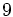# Direct product of M27 and Z3

View a complete list of particular groups (this is a very huge list!)[SHOW MORE]

## Definition

This group is defined as the direct product of the semidirect product of Z9 and Z3 (the unique non-abelian group of order$27$ and exponent$9$) and the cyclic group of order three.

## GAP implementation

### Group ID

This finite group has order 81 and has ID 13 among the groups of order 81 in GAP's SmallGroup library. For context, there are 15 groups of order 81. It can thus be defined using GAP's SmallGroup function as:

SmallGroup(81,13)

For instance, we can use the following assignment in GAP to create the group and name it$G$:

gap> G := SmallGroup(81,13);

Conversely, to check whether a given group$G$ is in fact the group we want, we can use GAP's IdGroup function:

IdGroup(G) = [81,13]

or just do:

IdGroup(G)

to have GAP output the group ID, that we can then compare to what we want.

### Other descriptions

The group can be described using GAP's DirectProduct, SmallGroup, and CyclicGroup functions:

DirectProduct(SmallGroup(27,4),CyclicGroup(3))